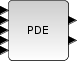Scilab Home page | Wiki | Bug tracker | Forge | Mailing list archives | ATOMS | File exchange
Change language to: English - Français - Português - Русский

# PDE

1D PDE block

### Block Screenshot### Description

This block is an implementation of several numerical schemes (Finite Elements (1st and 2nd order), Finite Differences (1st and 2nd order), Finite Volumes (1st order)) to solve mono-dimensional PDE (Partial Differential Equation) within Xcos. The mathematical framework is restricted to PDEs linear scalars with maximum order 2 in time and space. The goal is to provide engineers and physicists with an easy-to-use toolbox in Xcos that will let them graphically describe the PDE to be solved. A decision system selects the most efficient numerical scheme depending on the type of the PDE and runs the solver.

### Dialog box• Domain

a and b : (double) The two edges of the discretization field.

• PDE specification

• Check boxes to select the PDE operators. ai(x), bi(t) (i=1:7) are the operator coefficients.
• Discriminant type : constant or variable, in the later case, the sign should be given.
• Discretization method

• Choice (check box) : is the choice for the manual or the automatic mode.
• Type : in the manual mode we can give the method type (Finite differences, finite elements or finite volumes).
• Degree : method degree (1 or 2 for the FD and FE methods, 1 for the FV method).
• Number of nodes : to give the number of the nodal points.
• Initial Conditions

u(x,t0)=, du/dt at t0= : to give the initial conditions.

• Boundary condition on a

• Type : two types of boundary conditions are possible : Dirichlet or Neumann.
• Expression : to give the boundary conditions expression.
• Measurement points

To give the list of measurement points.

• Name

A getvalue box to give the block's name.

### Default properties

• always active: yes

• direct-feedthrough: no

• zero-crossing: no

• mode: no

• regular inputs:

- port 1 : size [1,1] / type 1

- port 2 : size [1,1] / type 0

- port 3 : size [1,1] / type 0

- port 4 : size [1,1] / type 0

- port 5 : size [1,1] / type 1

• regular outputs:

- port 1 : size [10,1] / type 1

- port 2 : size [0,1] / type 1

• number/sizes of activation inputs: 0

• number/sizes of activation outputs: 0

• continuous-time state: yes

• discrete-time state: no

• object discrete-time state: no

• name of computational function: PDE

### Interfacing function

• SCI/modules/scicos_blocks/macros/PDE/PDE.sci

### Computational functions

• SCI/modules/scicos_blocks/macros/PDE/